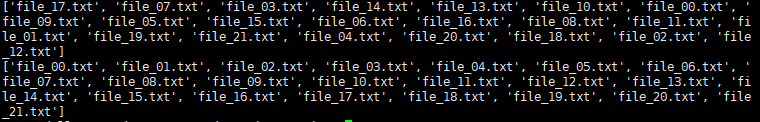# os 篇

### os.listdir(path)

import os

path = 'wuliytTaotao'
if not os.path.exists(path):
os.mkdir(path)

# 创建22个文件
for i in range(22):
f = open(os.path.join(path, 'file_%02d.txt' % i), 'w')
f.write(str(i))
f.close()

print(os.listdir(path))            # 在 windows 和 linux 上结果不一样，windows 上有序，而 linux 上无序
print(sorted(os.listdir(path)))    # 在 windows 和 linux 上排序后的结果一样


linux上的结果：# 运算符篇

## is vs. ==

is 判断两个对象是不是同一个 id，而 == 判断两个对象的内容是否相等。判断两个对象（int、str 等）的内容是否相等，用 == 更加保险。

### 实例 1：判断两个整数相等

a = 1
b = 1
a == b    # True
a is b    # True
c = 1234
d = 1234
c == d    # True
c is d    # False


### 实例 2：argparse 传参

import argparse

parser = argparse.ArgumentParser()

dest="boy", type=str, default="handsome")

args = parser.parse_args()
print(args)

boy = args.boy

print(boy is 'handsome')
print(boy == 'handsome')


### 实例 3：np.where

import numpy as np

a = np.array([True, False, False, True])

print(np.where(a == True))    # (array([0, 3]),)
print(np.where(a is True))    # (array([], dtype=int64),)


# 命令行参数篇

argparse 在解析 bool 参数时，如果按照 parser.add_argument('-a', dest='a', type=bool, default=True)解析参数 a，那么命令行中 -a False-a True 返回都是 True，而取不到 False。

posted @ 2019-07-05 10:34  wuliytTaotao  阅读(367)  评论(0编辑  收藏